# MCQs on Control Systems

##### Page 13 of 23. Go to page 1 2 3 4 5 6 7 8 9 10 11 12 13 14 15 16 17 18 19 20 21 22 23
01․ The impulse response of an initally relaxed linear system is e-2tu(t). To produce a response of te-2tu(t), the input must be equal to
e-tu(t).
e-2tu(t).
2e-tu(t).
(0.5)e-2tu(t).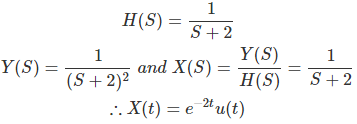02․ When the input to a system was with drawn at t = 0, its output was found to decrease exponentially from 1000 units to 500 units in 1.386 sec. The time constant of the system is
2.000.
1.386.
0.693.
0.500.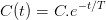C(o) = 1000 = C C(t) = 1000 e-t/T 500 = 1000 e-1.386/T ∴ T = 2 sec.

03․ The transfer function of a system is 10/(1 + S) when operated as a unity feedback system, the steady state error to a unit step input will be
Zero.
10.
1/11.
¾.

The given transfer function is to be taken as space open loop transfer function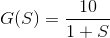This is a type zero system. The steady state error to a unit step input will be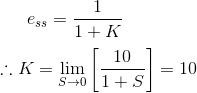⇒ ess = 1/11.

04․ The Laplace transformation of f(t) is F(S) given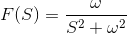the final value of f(t) is
None of them.
One.
Infinite.
Zero.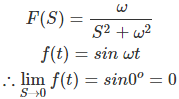05․ The position and velocity error coefficient for the transfer function given below are respectively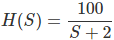0 and 0.
0 and infinity.
50 and 0.
50 and infinity.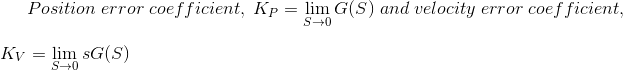06․ The device necessarily used for automatic temperature control in a furnace is
Thermostat.
Auto-transformer.
Thermocouple.
Any of above.

If the temperature is to be controlled automatically some form of thermostat should be used in the circuit so that is operates and switches out or switches in the oven whenever the temperature goes above or below a certain predetermined value respectively. The switching is to be carried out by contactors.

07․ Transient response is improved in case of
lag network.
both A and B.
none of these.

Basically a lead network has the improved transient response, good stability and reduced steady state error.

08․ The system is initially critical damped. If the gain of the system is doubled, then it will exhibit
over damped characteristics.
critical damped characteristics.
under damped characteristics.
undamped characteristics.

For critical damped system the value of damping factor (ξ) is unity. Generally ξ ∝ 1/√k. Where, k = system gain. Therefore, if the gain of the system is doubled, the value of damping factor will be less than unity and the system will exhibit under damped characteristics.

09․ The open loop transfer function of a unity feedback control system is given by G(s) = k/s(s+1). If the gain k is increased to infinity, then damping ratio will tend to become
zero
0.707
unity
infinite

Given open loop transfer function G(s) = k/s(s+1) Characteristic equation 1+G(s) = s²+s+k = 0 By comparing this characteristic equation with standard second order equation, Natural frequency ωn = √k Damping factor ξ = 1/(2√k) Therefore, if the gain k is increased to infinity, then damping ratio will tend to become zero.

10․ A proportional plus derivative controller
has high sensitivity
increases the stability of the system